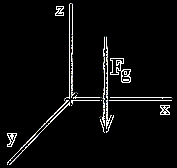﻿ 3D Projectile Motion | Zona Land Education

# Yet Another Projectile Motion Demonstration

Well, partially just for fun, here is a program demonstrating projectile motion in 3-D. Can you notice that accelerations only occur vertically, and that the horizontal velocities are constant?

See:

Spacing:

Above is a three dimensional rotating view of an object in ordinary projectile motion, no air resistance. The trajectory is drawn in white. Periodically along its flight a set of x, y, and z axes is drawn in red, green, and blue colors respectively. In this animation the z-axis is vertical. The x- and y-axes are horizontal.Of course, the force of gravity, or Fg, pulls downward. Thus projectile experiences a vertical force aimed downward along the z-direction. There are no forces pulling along the x- or y-directions. Therefore, the projectile will accelerate in the z-direction due to the pull of gravity. It will not accelerate in the x- or y-directions, since there are no forces in those directions.

Remember that when an acceleration occurs, a velocity must change. So, the projectile changes velocity in the z-direction. It does not change velocity in the x- or y-directions.

Notice that in the above demonstration the lines parallel to the z-axis, all colored blue, are spaced equally as time passes during the flight of the projectile. These axes mark the (x, y) motion of the projectile. There is no acceleration in the x- or the y-directions for this motion, and, therefore, there is no change in velocity in these directions.

So, the projectile travels through equal distances in equal time intervals along the x and y-directions. The resulting path that is projected on to the (x, y) plane is a straight line. The set of z lines (the blue lines) mark this linear path.

Custom Search